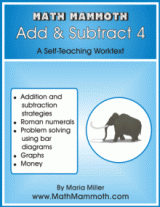### A new book for the Blue Series: Math Mammoth Add & Subtract 4

I have a new book to add to the Blue Series collection of worktexts:

Math Mammoth Add & Subtract 4Math Mammoth Add & Subtract 4 covers the following addition and subtraction related topics which are approximately for the third and fourth grade level:

* mental addition and subtraction strategies;
* adding and subtracting in columns;
* Roman numerals;
* the connection between addition and subtraction, missing addend problems, and solving word problems with bar diagrams;
* order of operations;
* bar graphs, line graphs, and money problems.

Samples pages include:

Contents and Introduction
More Subtraction Review
Subtract in Columns
Word Problems and Bar Diagrams
Rounding
Line Graphs